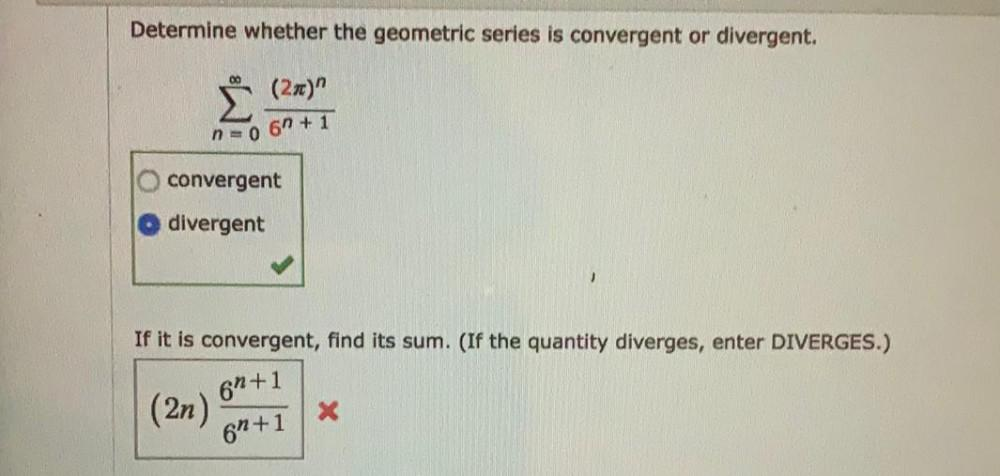Question:

# Determine whether the geometric series is convergent or divergent. 00 Ë (2x)" no 6n + 1 convergent divergent ) If it is convergeDetermine whether the geometric series is convergent or divergent. 00 Ë (2x)" no 6n + 1 convergent divergent ) If it is convergent, find its sum. (If the quantity diverges, enter DIVERGES.) 61+1 (2n) X 61+1